Circular Collision Detection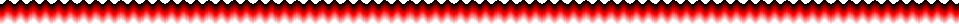This is a guide you can use to determine if two objects are intersecting, or have "collided."  This isn't the most efficient way of doing this.  If the shapes you are using are rectangular, there is a different (and more exact) way of determining if they are intersecting which you can find in my
VB Rectangular Collision Detection guide (working on it).

This guide works best for circular objects, and MAY work better then the Rectangular Collision guide for irregularly shaped objects.  If your program has objects that are circular though, this is the guide for that project.

All that you need to do to tell if there is a circular collision is to have the Central X and Central Y coordinates of an object, and the width and height of the object, Here is how you'd find the Central X and Central Y of an object with Left, Top, Width, and Height properties:

 Dim CircleX1 As Single, CircleY1 As Single Dim CircleX2 As Single, CircleY2 As Single CircleX1 = Object1.Left + Object1.Width / 2 CircleY1 = Object1.Top + Object1.Height / 2 CircleX2 = Object2.Left + Object2.Width / 2 CircleY2 = Object2.Top + Object2.Height / 2

The Central X and Central Y values that you get are the centers of imaginary circles that will surround your objects, where Object1 and Object2 are the two objects. The radius of the circle can be determined in whichever manner works best for your project.

1. You could use half either the width or height of the object for the radius, whichever is SMALLER, this way you can be certain that none of the object will be outside the circle, which will later translate into there being no collision at all unless that part of the object that the circle is over hits the other object, which would like like this :2. You could use half either the width or height of the object for the radius, whichever is BIGGER, this way you can be certain that none of the object will be outside the circle, which will later translate into there being a collision if almost any of the object intersects the other object :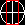3. OR you could use the average of #1 and #2, which would sometimes work better, it would look like this:This is how you would find the radius of the object using the three methods above.

Obviously the last option takes less code, so that's what I usually use, but you should try them out and see which one works best for whatever you're doing.  In general, as long as a game is going relatively fast, even if this isn't perfect it will work for you.

So now, You have two circles, with a central point, and a radius: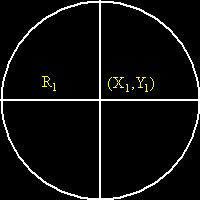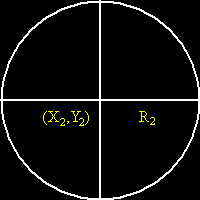For now we'll ignore the circles for a second and just look at the centers of them.  The key to this is to find how far apart the center of the two objects are.  Which requires a little bit of math.  The triangle that's formed you can see below.The two acute angles of the triangle have vertexes at the centers of the two objects, the point at the right angle creates a right triangle.  The length of the two sides are the differences between the two X values, and the two Y values.  Now if you use the Pythagorean theorem [A2 + B2 = C2], you can find the hypotenuse, which is the length between the centers.  To do this in code:

 Dim DifY As Single, DifX As Single Dim Distance As Single DifY = CircleY1 - CircleY2 DifX = CirlcleX1 - CircleX2 Distance = Sqr(DifY ^ 2 + DifX ^ 2)
• Where CircleY1, CircleY2, CirlcleX1, and CircleX2 came from the above code.  Now you have the Distance between the two centers, how is that useful you may ask.  I'm not exactly sure how to describe this in words, so first I'll show you two pictures, the left of two objects (circles, like we would have found above) that aren't intersecting, the right of two objects (circles) that are intersecting.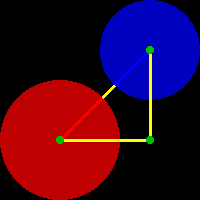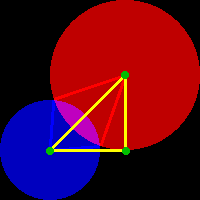So how do we tell if we have the left or the right going on, in code?  Well we have to compare the sum of the two circles radii with the distance between there centers.  If the sum of the radii is less then the distance between the centers of the two circles, then there is no intersection, as you can see in the left picture.  If the sum of the radii is greater then the distance between the centers of the two circles, then the circles ARE intersecting, as you can see in the right picture.  Here is how you would tell using code:

Where CircleRadius1, CircleRadius2, and Distance are from above.  Now we can put it all together to get the final check (We'll use Method 3 for finding the radius):

 ~~